# New to QlikView

Discussion board where members can get started with QlikView.

Announcements
Modernize Your QlikView Deployment webinar, Nov. 3rd. REGISTER
cancel
Showing results for
Did you mean:
HighlightedCreator

## Help with Invoice equal to zero

Hello community!!

I am currently working on a dashboard that allows me to identify invoices that are equal to zero.
I have noticed the existence of some charges in the invoices that not in all cases is correct to be billed as "shipping cost invoice" and "delinquency cost". Then I should be able to calculate two KPI'S:

1) when the invoices are equal to zero.
2) and when the sum of "Total Invoive Charge" in field
INVOICE_NUM is equal to zero, assuming there are no charges: "shipping cost invoice" and "delinquency cost"

Point 2) will help me establish more precisely the service charges that really are zero.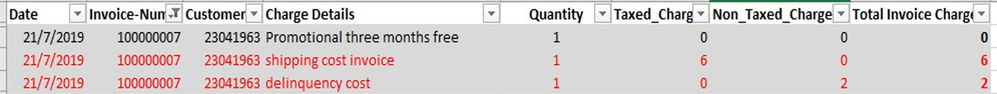The previous example shows a case that when adding the threes charges of the invoice the total is 8, but really the charge is zero, since it includes the items not to be considered for the analysis.

The third requirement is not a KPI, but I would like to be able to write a field from the script in order to have the "Total Amount of the Invoice". Currently the data brings the total but at the level of charge.

Finally, I have included a ready list related to point 1) and allow filtering the cases with invoices equal to zero, with the following expression: = Aggr (if (sum ([Total Invoice Charge]) = 0, 'Invoice Equal to zero ',' N / A '), [Invoice-Num]). I would like those cases only to be in a pivot chart (try it but it shows me the rest of the cases) and also in a bar chart.

Thanks again, counting on your usual help

3 Solutions

Accepted Solutions
HighlightedMVP

Try this

``=Count(DISTINCT {<[Invoice-Num] = {"=Sum({<[Charge Details] = {'delinquency cost'}>} [Total Invoice Charge]) > 0 and Sum({<[Charge Details] = {'shipping cost invoice'}>} [Total Invoice Charge]) > 0 and Sum({<[Charge Details] -= {'shipping cost invoice', 'delinquency cost'}>} [Total Invoice Charge]) = 0"}>} [Invoice-Num])``
HighlightedMVP

Try this

``=Count(DISTINCT {<[Invoice-Num] = {"=(Sum({<[Charge Details] = {'delinquency cost'}>} [Total Invoice Charge]) > 0 or Sum({<[Charge Details] = {'shipping cost invoice'}>} [Total Invoice Charge]) > 0) and Sum({<[Charge Details] -= {'shipping cost invoice', 'delinquency cost'}>} [Total Invoice Charge]) = 0"}>} [Invoice-Num])``
HighlightedMVP

I think my expression is already excluding when the amount  = 0... I reloaded with the new file attached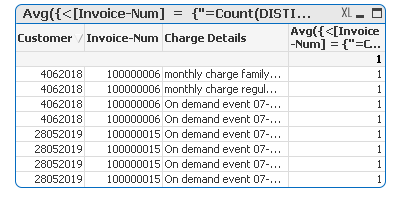.

For a distinct count of Invoice Number, you can try this

``=Count(DISTINCT {<[Invoice-Num] = {"=Count(DISTINCT [Charge Details]) > 1 and Max(Aggr(Count({<Taxed_Charge = {[<>0]}>}Taxed_Charge), [Invoice-Num], Taxed_Charge)) > 1 "}>} [Invoice-Num])``

For overall count

``=Count({<[Invoice-Num] = {"=Count(DISTINCT [Charge Details]) > 1 and Max(Aggr(Count({<Taxed_Charge = {[<>0]}>}Taxed_Charge), [Invoice-Num], Taxed_Charge)) > 1 "}>} [Invoice-Num])``

12 Replies
HighlightedCreator

Hi! after some tests and better define the need, which is definitely:
1) Get the Invoice-Num where the charges exist: "shipping cost invoice" and "delinquency cost".
2) Once these Invoice-Num are obtained, to be able to identify the other charges when they are equal to zero.

For the first case, use the p () function in a list box as follows:

=Aggr(Only({1<[Invoice-Num] = p({1<[Charge Details] = {'delinquency cost', 'shipping cost invoice'}>}[Invoice-Num])>} [Invoice-Num]), [Invoice-Num])

For the second case, use the following expression in another list box:
= Aggr (if (sum ([Total Invoice Charge]) = 0, 'Charge Details - Equal to zero', 'N / A'), [Charge Details])

The above can work for me but I would like to simplify it in a single filter and not depend on two list boxes, I also need to be able to transfer the result to a pivot chart without having to depend on list boxes, so that everything is summarized in a table... I don't know how to get it

HighlightedMVP

I am not sure I understand completely, would you be able to provide the output you are expecting to see from the sample you have shared?

HighlightedCreator

I attach the data file (The qvw. file is the same on the previous post).

The result I hope is to be able a expression to count when: in a "x" invoice (A), there are certain charges (Shipping cost invoice or delinquency cost) (B) on an invoice, and another "X" charge is equal to zero (C).

In the following image I explain the case I want to be able to count.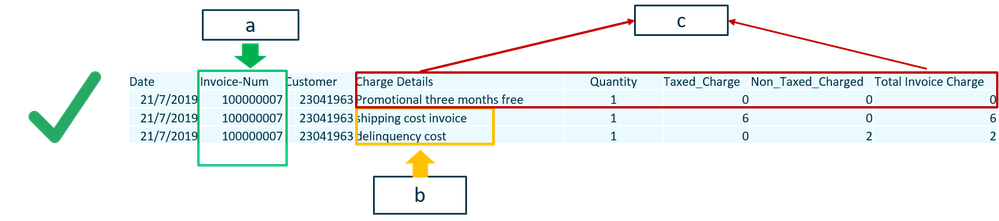The cases that I am not interested in identifying, nor counting are the following:In the previous example, the other charge different than "Shipping cost invoice or delinquency cost" is > 0, for dashboard functionality it is not the objective to measure it.

Another example of result not desired is:In the previous example, although there is a charge other than Shipping cost or delinquency cost equal to zero, there is another charge that is> 0, so the objective condition to measure it is not met.

I hope you understand, as always thanks for your help!

HighlightedMVP

Try this

``=Count(DISTINCT {<[Invoice-Num] = {"=Sum({<[Charge Details] = {'delinquency cost'}>} [Total Invoice Charge]) > 0 and Sum({<[Charge Details] = {'shipping cost invoice'}>} [Total Invoice Charge]) > 0 and Sum({<[Charge Details] -= {'shipping cost invoice', 'delinquency cost'}>} [Total Invoice Charge]) = 0"}>} [Invoice-Num])``
HighlightedCreator

Thanks sunny! @sunny_talwar  Solve my need for now..

although seeing it well, look at the case of invoice 100000005, where only one of the conditions (shipping cost invoice) is included. The expression you suggested is omitting that record.

That case would also be OK for the analysis, look:That is, one or two of the conditions may exist, it should not necessarily be restricted to the existence of both invoices ('delinquency cost', 'shipping cost invoice') You see it?

HighlightedMVP

Try this

``=Count(DISTINCT {<[Invoice-Num] = {"=(Sum({<[Charge Details] = {'delinquency cost'}>} [Total Invoice Charge]) > 0 or Sum({<[Charge Details] = {'shipping cost invoice'}>} [Total Invoice Charge]) > 0) and Sum({<[Charge Details] -= {'shipping cost invoice', 'delinquency cost'}>} [Total Invoice Charge]) = 0"}>} [Invoice-Num])``
HighlightedCreator

It works perfect!! You're a genious!!! 😃

Thanks!

HighlightedCreator

I found something extra in the invoices to be solved, I have got "Charge Details" that seem to be duplicated. I wish I could in an expression count when on an invoice:

1. There is more than one charge with the same amount,
2. And charge details may not be the same.

Something like this: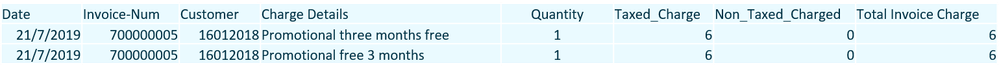You can see that within the same invoice there are at least 2 charges for the same amount (Taxed_Charge), even if they are called different. In essence it should be a single charge and double billed.

Any help would be greatly appreciated!

HighlightedMVP

Like this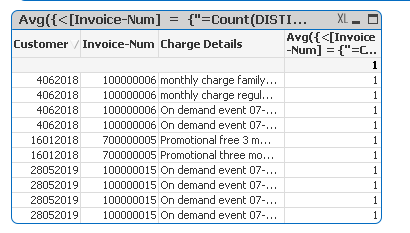Expression

``Avg({<[Invoice-Num] = {"=Count(DISTINCT [Charge Details]) > 1 and Max(Aggr(Count({<Taxed_Charge = {[<>0]}>}Taxed_Charge), [Invoice-Num], Taxed_Charge)) > 1 "}>} 1)``